# Control Statements Spring Semester 2013 Programming and Data

• Slides: 58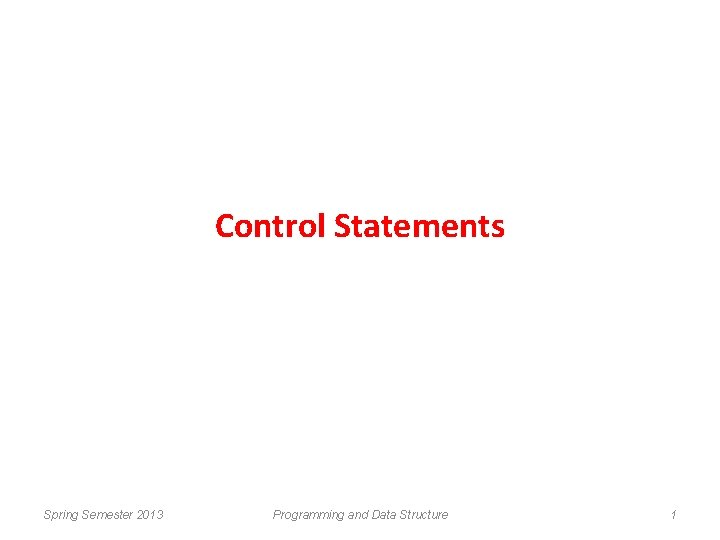Control Statements Spring Semester 2013 Programming and Data Structure 1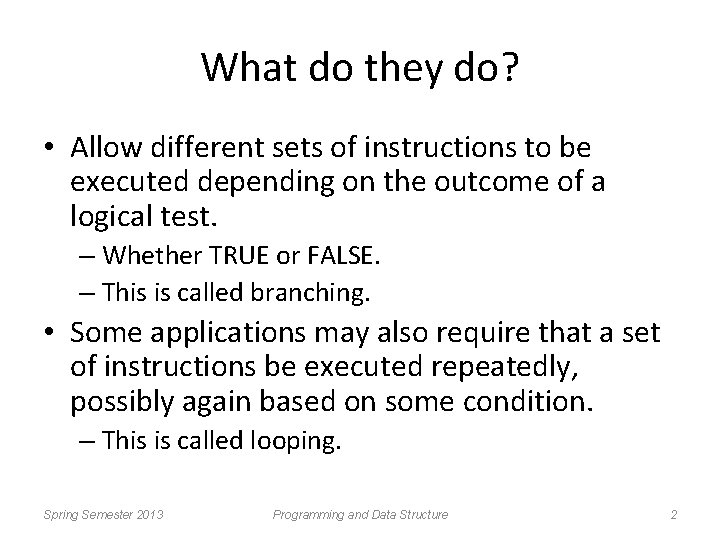What do they do? • Allow different sets of instructions to be executed depending on the outcome of a logical test. – Whether TRUE or FALSE. – This is called branching. • Some applications may also require that a set of instructions be executed repeatedly, possibly again based on some condition. – This is called looping. Spring Semester 2013 Programming and Data Structure 2How do we specify the conditions? • Using relational operators. – Four relation operators: – Two equality operations: <, <=, >, >= ==, != • Using logical operators / connectives. – Two logical connectives: – Unary negation operator: Spring Semester 2013 Programming and Data Structure &&, | | ! 3Examples count <= 100 (math+phys+chem)/3 >= 60 (sex==‘M’) && (age>=21) (marks>=80) && (marks<90) (balance>5000) | | (no_of_trans>25) ! (grade==‘A’) ! ((x>20) && (y<16)) Spring Semester 2013 Programming and Data Structure 4The conditions evaluate to … • Zero – Indicates FALSE. • Non-zero – Indicates TRUE. – Typically the condition TRUE is represented by the value ‘ 1’. Spring Semester 2013 Programming and Data Structure 5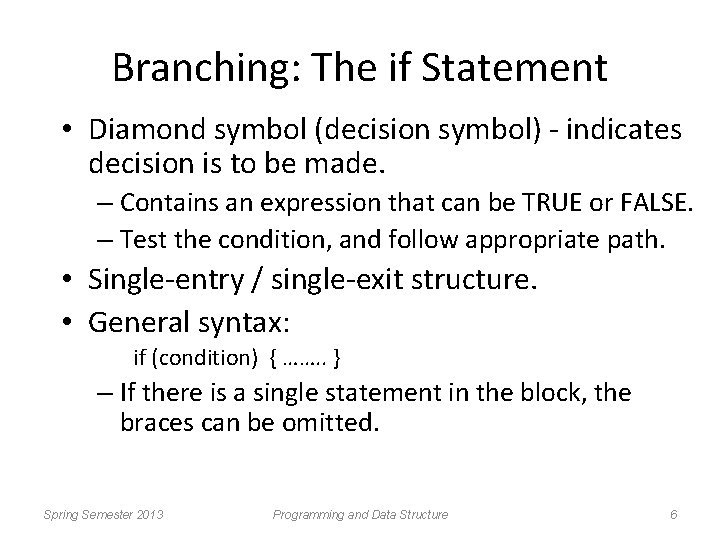Branching: The if Statement • Diamond symbol (decision symbol) - indicates decision is to be made. – Contains an expression that can be TRUE or FALSE. – Test the condition, and follow appropriate path. • Single-entry / single-exit structure. • General syntax: if (condition) { ……. . } – If there is a single statement in the block, the braces can be omitted. Spring Semester 2013 Programming and Data Structure 6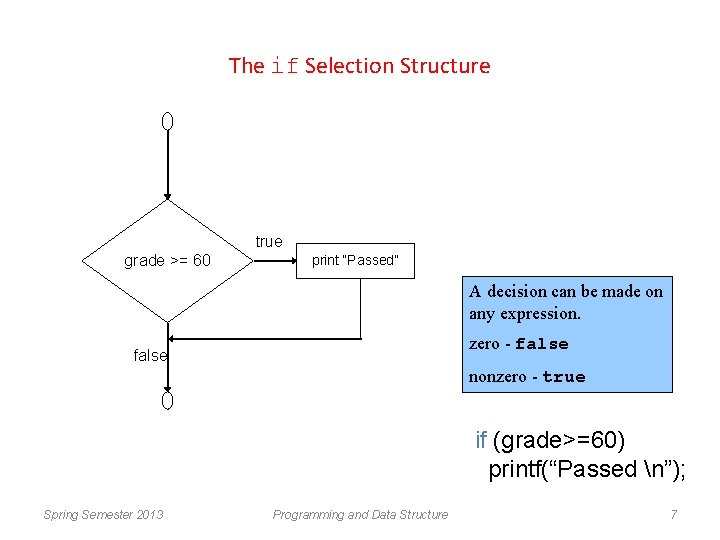The if Selection Structure true grade >= 60 print “Passed” A decision can be made on any expression. zero - false nonzero - true if (grade>=60) printf(“Passed n”); Spring Semester 2013 Programming and Data Structure 7Example #include <stdio. h> main() { int a, b, c; scanf (“%d %d %d”, &a, &b, &c); if ((a>=b) && (a>=c)) printf (“n The largest number is: %d”, a); if ((b>=a) && (b>=c)) printf (“n The largest number is: %d”, b); if ((c>=a) && (c>=b)) printf (“n The largest number is: %d”, c); } Spring Semester 2013 Programming and Data Structure 8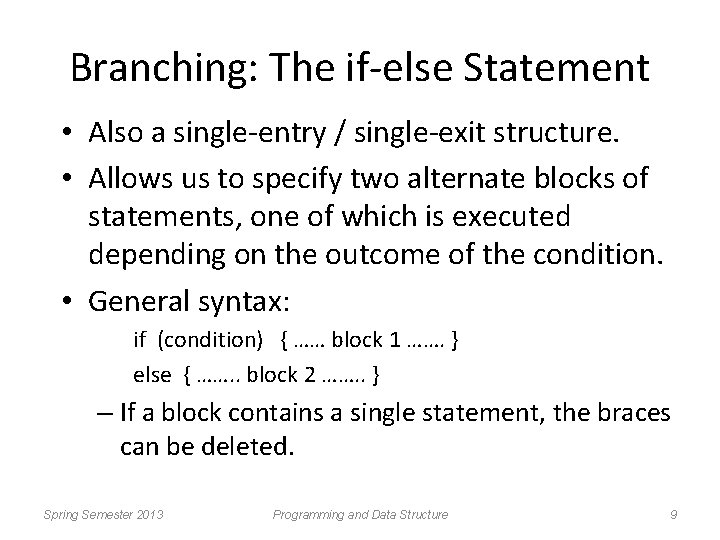Branching: The if-else Statement • Also a single-entry / single-exit structure. • Allows us to specify two alternate blocks of statements, one of which is executed depending on the outcome of the condition. • General syntax: if (condition) { …… block 1 ……. } else { ……. . block 2 ……. . } – If a block contains a single statement, the braces can be deleted. Spring Semester 2013 Programming and Data Structure 9The if/else Selection Structure false grade >= 60 true print “Passed” print “Failed” if ( grade >= 60 ) printf( "Passedn"); else printf( "Failedn"); Spring Semester 2013 Programming and Data Structure 10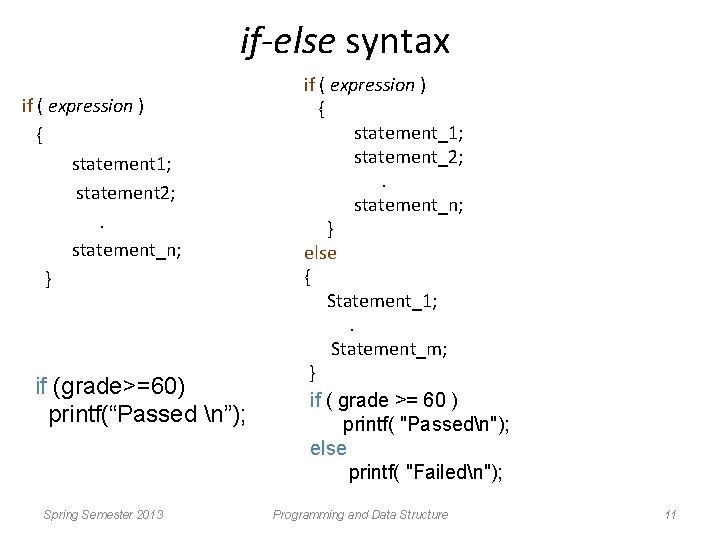if-else syntax if ( expression ) { statement 1; statement 2; . statement_n; } if (grade>=60) printf(“Passed n”); Spring Semester 2013 if ( expression ) { statement_1; statement_2; . statement_n; } else { Statement_1; . Statement_m; } if ( grade >= 60 ) printf( "Passedn"); else printf( "Failedn"); Programming and Data Structure 11Nesting of if-else Structures • It is possible to nest if-else statements, one within another. • All if statements may not be having the “else” part. – Confusion? ? • Rule to be remembered: – An “else” clause is associated with the closest preceding unmatched “if”. Spring Semester 2013 Programming and Data Structure 12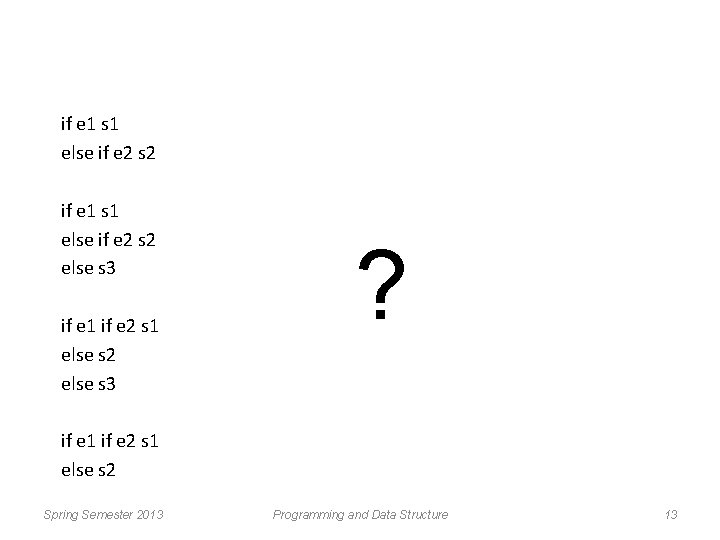if e 1 s 1 else if e 2 s 2 else s 3 if e 1 if e 2 s 1 else s 2 else s 3 ? if e 1 if e 2 s 1 else s 2 Spring Semester 2013 Programming and Data Structure 13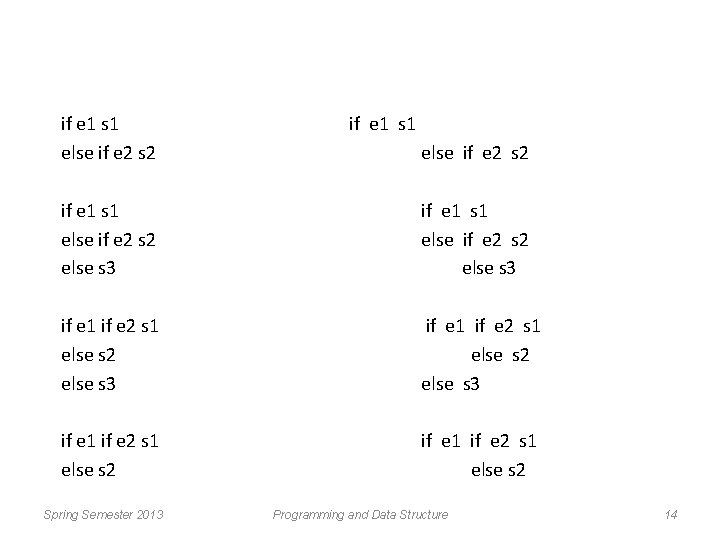if e 1 s 1 else if e 2 s 2 else s 3 if e 1 if e 2 s 1 else s 2 Spring Semester 2013 Programming and Data Structure 14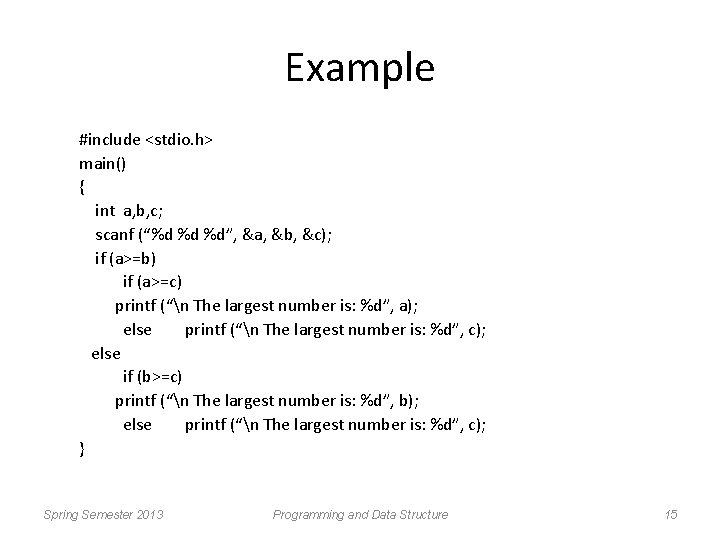Example #include <stdio. h> main() { int a, b, c; scanf (“%d %d %d”, &a, &b, &c); if (a>=b) if (a>=c) printf (“n The largest number is: %d”, a); else printf (“n The largest number is: %d”, c); else if (b>=c) printf (“n The largest number is: %d”, b); else printf (“n The largest number is: %d”, c); } Spring Semester 2013 Programming and Data Structure 15Example #include <stdio. h> main() { int a, b, c; scanf (“%d %d %d”, &a, &b, &c); if ((a>=b) && (a>=c)) printf (“n The largest number is: %d”, a); else if (b>c) printf (“n The largest number is: %d”, b); else printf (“n The largest number is: %d”, c); } Spring Semester 2013 Programming and Data Structure 16Confusing Equality (==) and Assignment (=) Operators • Dangerous error – Does not ordinarily cause syntax errors – Any expression that produces a value can be used in control structures – Nonzero values are true, zero values are false • Example: if ( pay. Code == 4 ) printf( "You get a bonus!n" ); Checks paycode, if it is 4 then a bonus is awarded Equality check improper if ( pay. Code = 4 ) printf( "You get a bonus!n" ); Spring Semester 2013 Equality check proper if ( pay. Code == 4 ) printf( "You get a bonus!n" ); Programming and Data Structure 18Generalization of expression evaluation in C • Assignment (=) operation is also a part of expression. Returns the value 3 after assigning it to i. i=3; int i=4, j ; if(i=3) j=0; else j=1; Spring Semester 2013 j? int i=4, j ; if(i==3) j=0; Whatever be the value of i, else j is always 0. j=1; Programming and Data Structure j=1 19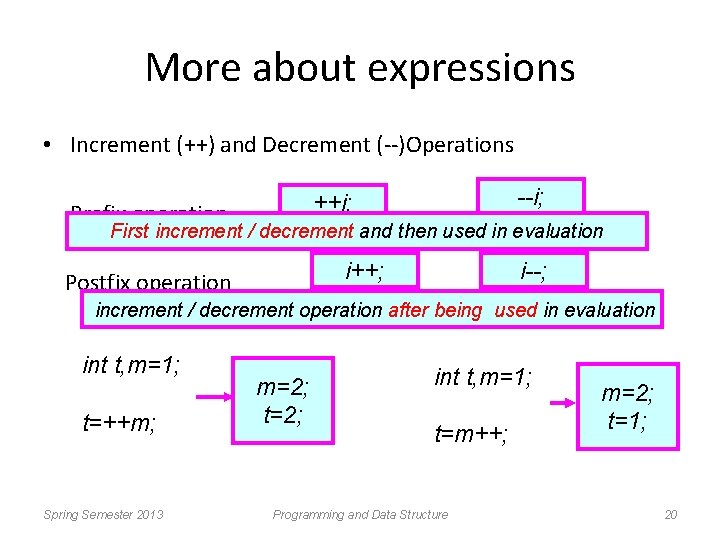More about expressions • Increment (++) and Decrement (--)Operations --i; ++i; Prefix operation First increment / decrement and then used in evaluation i++; Postfix operation i--; increment / decrement operation after being used in evaluation int t, m=1; t=++m; Spring Semester 2013 m=2; t=2; int t, m=1; t=m++; Programming and Data Structure m=2; t=1; 20Some More Examples Initial values : : a = 10; b = 20; x = 50 + ++a; a = 11, x = 61 x = 50 + a++; x = 60, a = 11 x = a++ + --b; b = 19, x = 29, a = 11 x = a++ – ++a; Spring Semester 2013 Undefined value (implementation dependent) Programming and Data Structure 21Ternary conditional operator (? : ) – Takes three arguments (condition, value if true, value if false) – Returns the evaluated value accordingly. grade >= 60 ? printf( “Passedn” ) : printf( “Failedn” ); (expr 1)? (expr 2): (expr 3); Example: interest = (balance>5000) ? balance*0. 2 : balance*0. 1; Returns a value Spring Semester 2013 Programming and Data Structure 22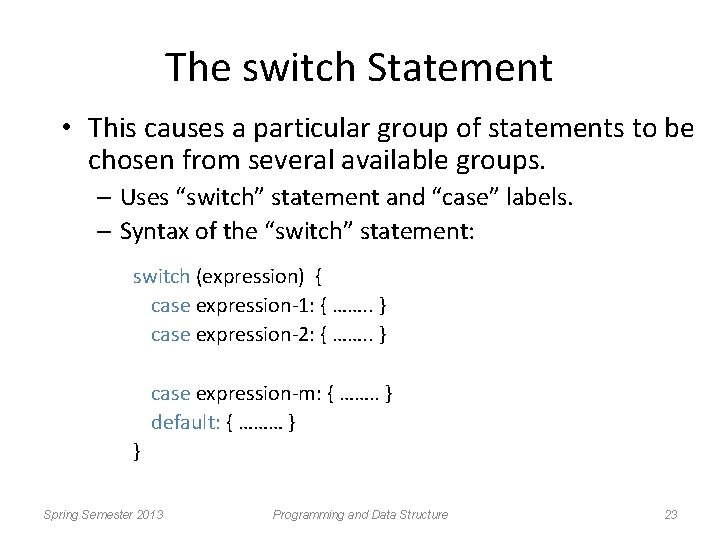The switch Statement • This causes a particular group of statements to be chosen from several available groups. – Uses “switch” statement and “case” labels. – Syntax of the “switch” statement: switch (expression) { case expression-1: { ……. . } case expression-2: { ……. . } case expression-m: { ……. . } default: { ……… } } Spring Semester 2013 Programming and Data Structure 23The switch Multiple-Selection Structure case a true case a action(s) break case b action(s) break case z action(s) break false case b true false. . . case z true false default action(s) Spring Semester 2013 Programming and Data Structure 24Example switch ( letter ) { case 'A': printf("First lettern"); break; case 'Z': printf("Last lettern"); break; default : printf("Middle lettern"); break; } Spring Semester 2013 Programming and Data Structure 25Example switch (choice = toupper(getchar())) { case ‘R’: case ‘G’: case ‘B’: default: printf (“RED n”); break; printf (“GREEN n”); break; printf (“BLUE n”); break; printf (“Invalid choice n”); } Spring Semester 2013 Programming and Data Structure 26Example switch (choice = getchar()) { case ‘r’: case ‘R’: case ‘g’: case ‘G’: case ‘b’: case ‘B’: default: printf (“RED n”); break; printf (“GREEN n”); break; printf (“BLUE n”); break; printf (“Invalid choice n”); } Spring Semester 2013 Programming and Data Structure 28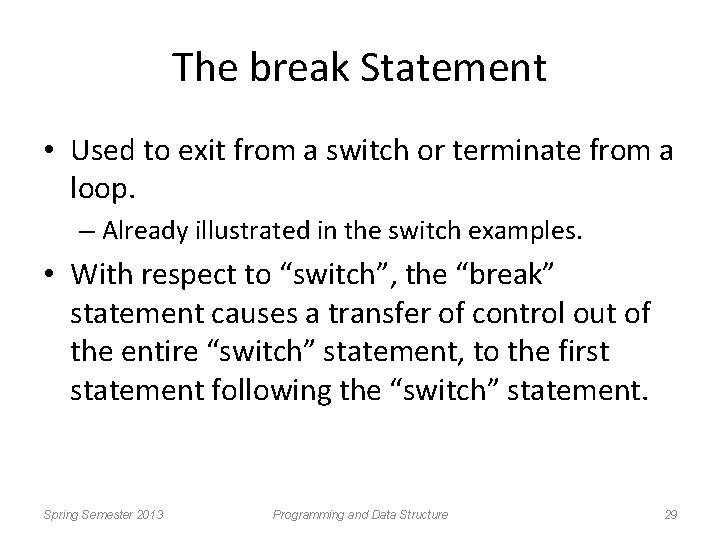The break Statement • Used to exit from a switch or terminate from a loop. – Already illustrated in the switch examples. • With respect to “switch”, the “break” statement causes a transfer of control out of the entire “switch” statement, to the first statement following the “switch” statement. Spring Semester 2013 Programming and Data Structure 29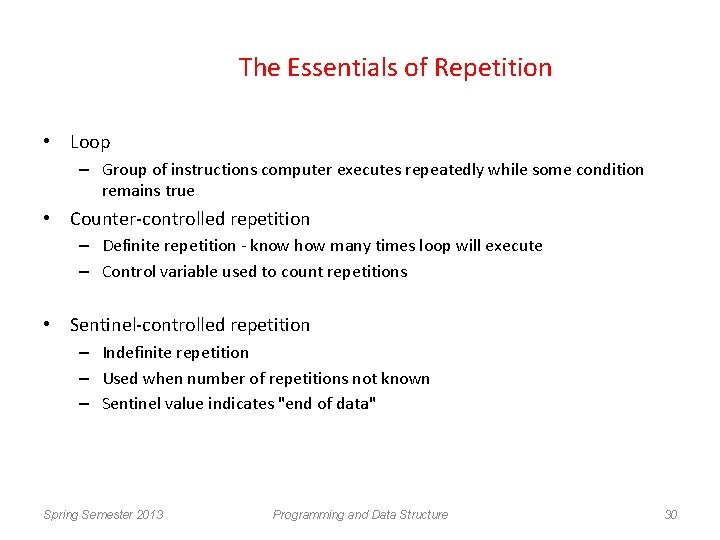The Essentials of Repetition • Loop – Group of instructions computer executes repeatedly while some condition remains true • Counter-controlled repetition – Definite repetition - know how many times loop will execute – Control variable used to count repetitions • Sentinel-controlled repetition – Indefinite repetition – Used when number of repetitions not known – Sentinel value indicates "end of data" Spring Semester 2013 Programming and Data Structure 30Counter-Controlled Repetition • Counter-controlled repetition requires – name of a control variable (or loop counter). – initial value of the control variable. – condition that tests for the final value of the control variable (i. e. , whether looping should continue). – increment (or decrement) by which the control variable is modified each time through the loop. int counter =1; //initialization while (counter <= 10) { //repetition condition printf( "%dn", counter ); ++counter; //increment } Spring Semester 2013 Programming and Data Structure 31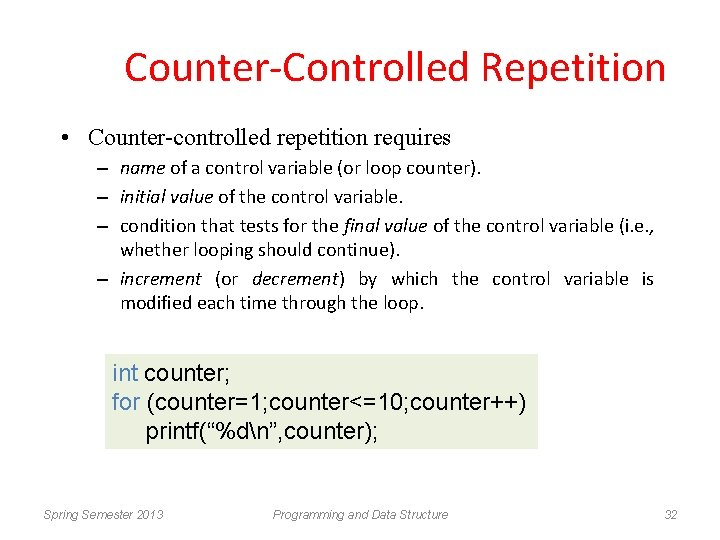Counter-Controlled Repetition • Counter-controlled repetition requires – name of a control variable (or loop counter). – initial value of the control variable. – condition that tests for the final value of the control variable (i. e. , whether looping should continue). – increment (or decrement) by which the control variable is modified each time through the loop. int counter; for (counter=1; counter<=10; counter++) printf(“%dn”, counter); Spring Semester 2013 Programming and Data Structure 32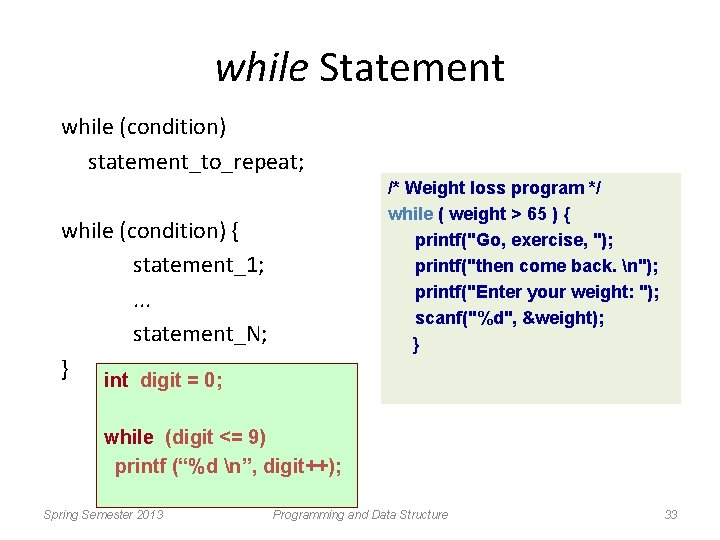while Statement while (condition) statement_to_repeat; /* Weight loss program */ while ( weight > 65 ) { printf("Go, exercise, "); printf("then come back. n"); printf("Enter your weight: "); scanf("%d", &weight); } while (condition) { statement_1; . . . statement_N; } int digit = 0; while (digit <= 9) printf (“%d n”, digit++); Spring Semester 2013 Programming and Data Structure 33false C true Single-entry / single-exit structure statement(s) Spring Semester 2013 Programming and Data Structure 34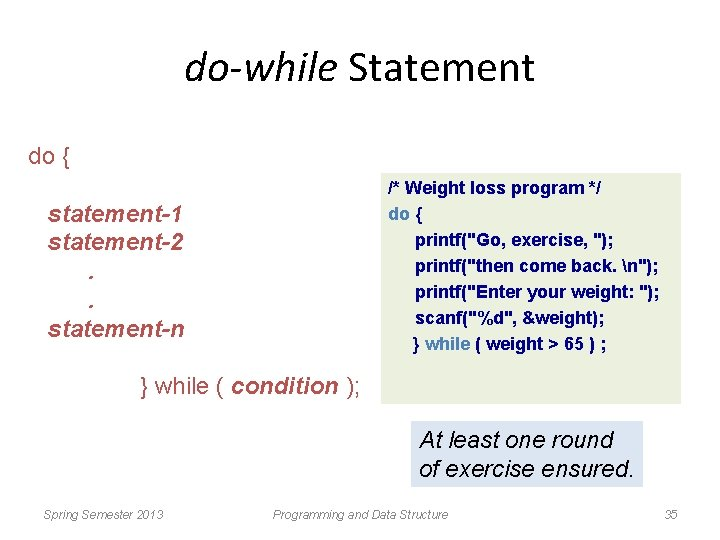do-while Statement do { /* Weight loss program */ do { printf("Go, exercise, "); printf("then come back. n"); printf("Enter your weight: "); scanf("%d", &weight); } while ( weight > 65 ) ; statement-1 statement-2. . statement-n } while ( condition ); At least one round of exercise ensured. Spring Semester 2013 Programming and Data Structure 35statement(s) Single-entry / single-exit structure false C true int digit = 0; do printf (“%d n”, digit++); while (digit <= 9); Spring Semester 2013 Programming and Data Structure 36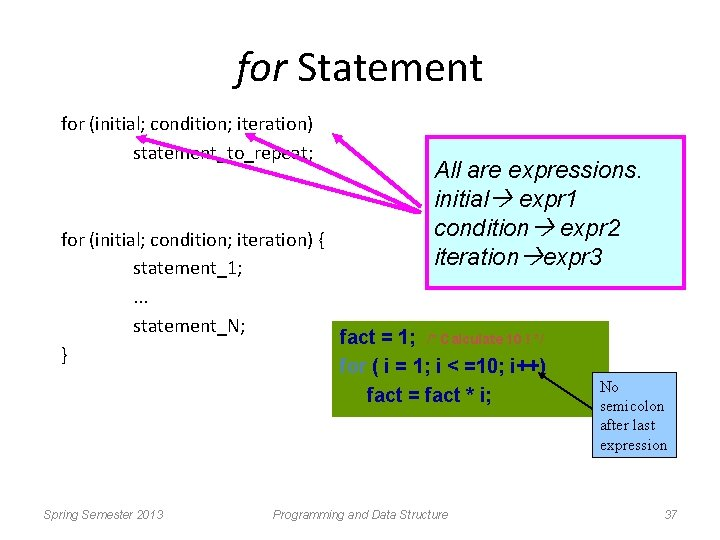for Statement for (initial; condition; iteration) statement_to_repeat; All are expressions. initial expr 1 condition expr 2 iteration expr 3 for (initial; condition; iteration) { statement_1; . . . statement_N; fact = 1; /* Calculate 10 ! */ } for ( i = 1; i < =10; i++) fact = fact * i; Spring Semester 2013 Programming and Data Structure No semicolon after last expression 37• How it works? – “expression 1” is used to initialize some variable (called index) that controls the looping action. – “expression 2” represents a condition that must be true for the loop to continue. – “expression 3” is used to alter the value of the index initially assigned by “expression 1”. int digit; for (digit=0; digit<=9; digit++) printf (“%d n”, digit); Spring Semester 2013 Programming and Data Structure 38• How it works? – “expression 1” is used to initialize some variable (called index) that controls the looping action. – “expression 2” represents a condition that must be true for the loop to continue. – “expression 3” is used to alter the value of the index initially assigned by “expression 1”. Spring Semester 2013 Programming and Data Structure 39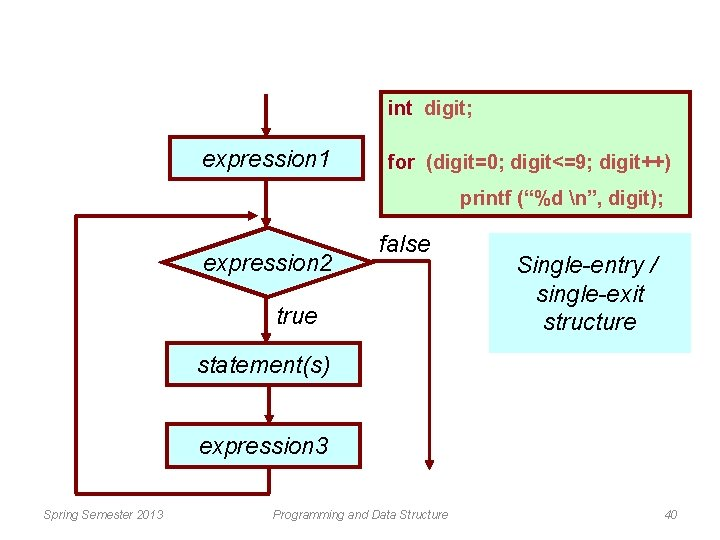int digit; expression 1 for (digit=0; digit<=9; digit++) printf (“%d n”, digit); expression 2 false true Single-entry / single-exit structure statement(s) expression 3 Spring Semester 2013 Programming and Data Structure 40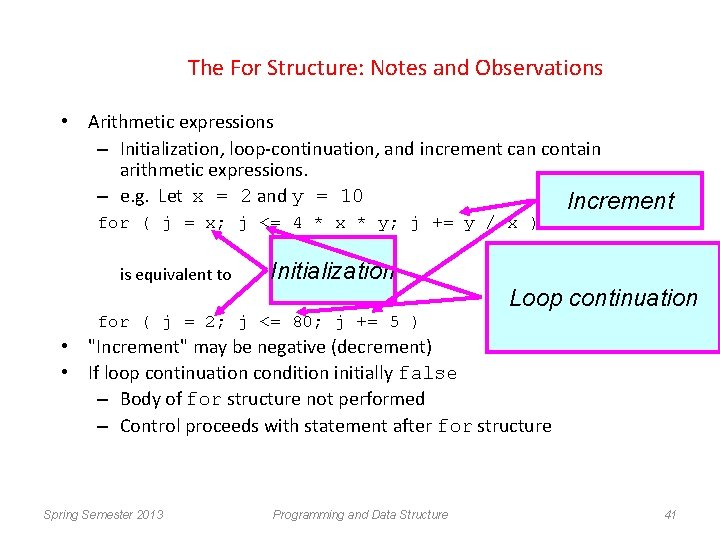The For Structure: Notes and Observations • Arithmetic expressions – Initialization, loop-continuation, and increment can contain arithmetic expressions. – e. g. Let x = 2 and y = 10 Increment for ( j = x; j <= 4 * x * y; j += y / x ) is equivalent to Initialization Loop continuation for ( j = 2; j <= 80; j += 5 ) • "Increment" may be negative (decrement) • If loop continuation condition initially false – Body of for structure not performed – Control proceeds with statement after for structure Spring Semester 2013 Programming and Data Structure 41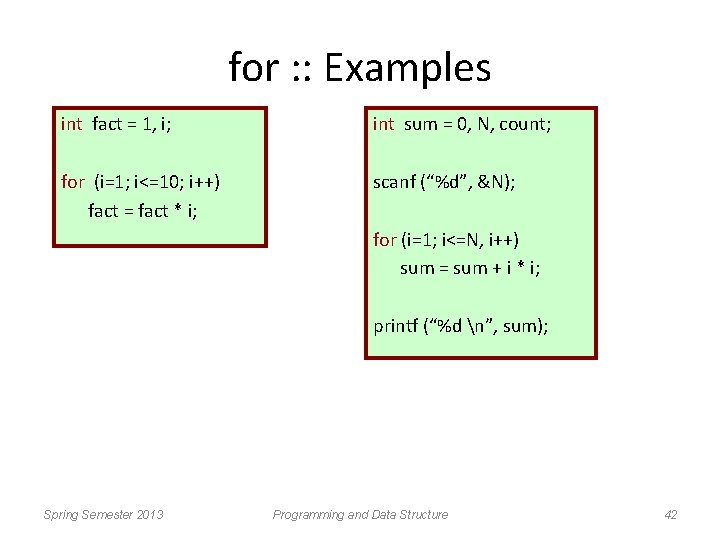for : : Examples int fact = 1, i; int sum = 0, N, count; for (i=1; i<=10; i++) fact = fact * i; scanf (“%d”, &N); for (i=1; i<=N, i++) sum = sum + i * i; printf (“%d n”, sum); Spring Semester 2013 Programming and Data Structure 42• The comma operator – We can give several statements separated by commas in place of “expression 1”, “expression 2”, and “expression 3”. for (fact=1, i=1; i<=10; i++) fact = fact * i; for (sum=0, i=1; i<=N, i++) sum = sum + i * i; Spring Semester 2013 Programming and Data Structure 43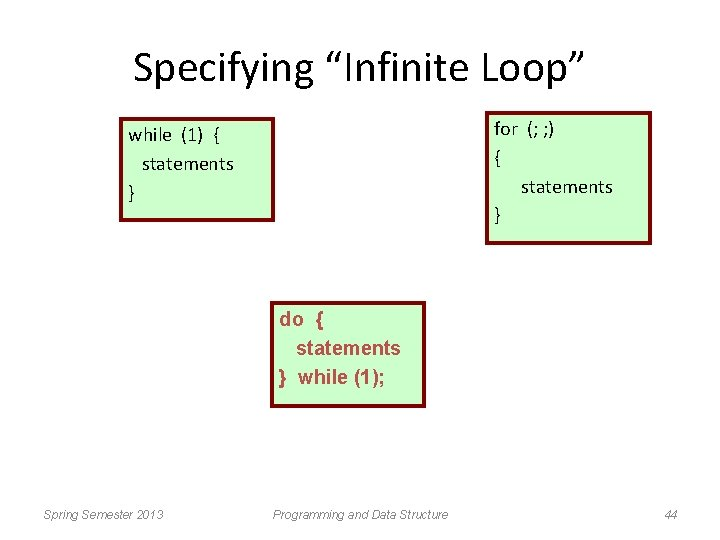Specifying “Infinite Loop” for (; ; ) { statements } while (1) { statements } do { statements } while (1); Spring Semester 2013 Programming and Data Structure 44break Statement • Break out of the loop { } – can use with • while • do while • for • switch – does not work with • if {} • else {} Causes immediate exit from a while, for, do/while or switch structure Program execution continues with the first statement after the structure Common uses of the break statement Escape early from a loop Skip the remainder of a switch structure Spring Semester 2013 Programming and Data Structure 45A Complete Example #include <stdio. h> main() { int fact, i; fact = 1; i = 1; } while ( i<10 ) { /* run loop –break when fact >100*/ fact = fact * i; if ( fact > 100 ) { printf ("Factorial of %d above 100", i); break; /* break out of the while loop */ } i ++ ; } Spring Semester 2013 Programming and Data Structure 46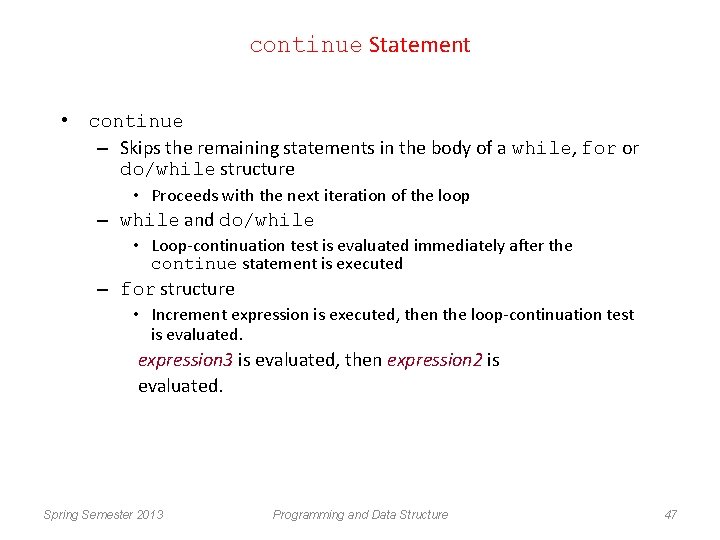continue Statement • continue – Skips the remaining statements in the body of a while, for or do/while structure • Proceeds with the next iteration of the loop – while and do/while • Loop-continuation test is evaluated immediately after the continue statement is executed – for structure • Increment expression is executed, then the loop-continuation test is evaluated. expression 3 is evaluated, then expression 2 is evaluated. Spring Semester 2013 Programming and Data Structure 47An Example with “break” & “continue” fact = 1; i = 1; while (1) { fact = fact * i; i ++ ; if ( i<10 ) continue; /* a program to calculate 10 ! /* not done yet ! Go to loop and perform next iteration*/ break; } Spring Semester 2013 Programming and Data Structure 48Some Examples Spring Semester 2013 Programming and Data Structure 49Example 1: Test if a number is prime or not #include <stdio. h> main() { int n, i=2; scanf (“%d”, &n); while (i < n) { if (n % i == 0) { printf (“%d is not a prime n”, n); exit; } i++; } printf (“%d is a prime n”, n); } Spring Semester 2013 Programming and Data Structure 50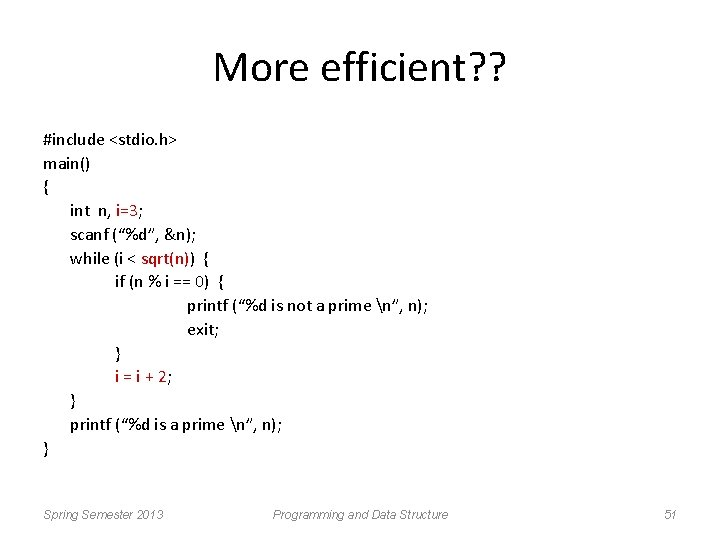More efficient? ? #include <stdio. h> main() { int n, i=3; scanf (“%d”, &n); while (i < sqrt(n)) { if (n % i == 0) { printf (“%d is not a prime n”, n); exit; } i = i + 2; } printf (“%d is a prime n”, n); } Spring Semester 2013 Programming and Data Structure 51Example 2: Find the sum of digits of a number #include <stdio. h> main() { int n, sum=0; scanf (“%d”, &n); while (n != 0) { sum = sum + (n % 10); n = n / 10; } printf (“The sum of digits of the number is %d n”, sum); } Spring Semester 2013 Programming and Data Structure 52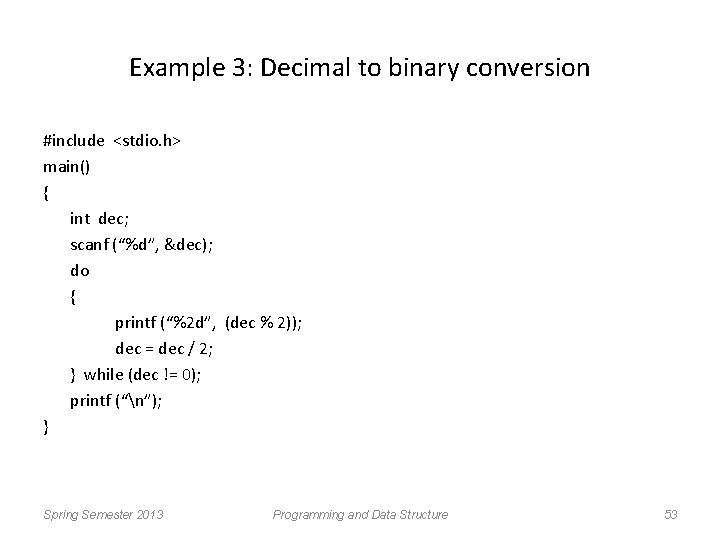Example 3: Decimal to binary conversion #include <stdio. h> main() { int dec; scanf (“%d”, &dec); do { printf (“%2 d”, (dec % 2)); dec = dec / 2; } while (dec != 0); printf (“n”); } Spring Semester 2013 Programming and Data Structure 53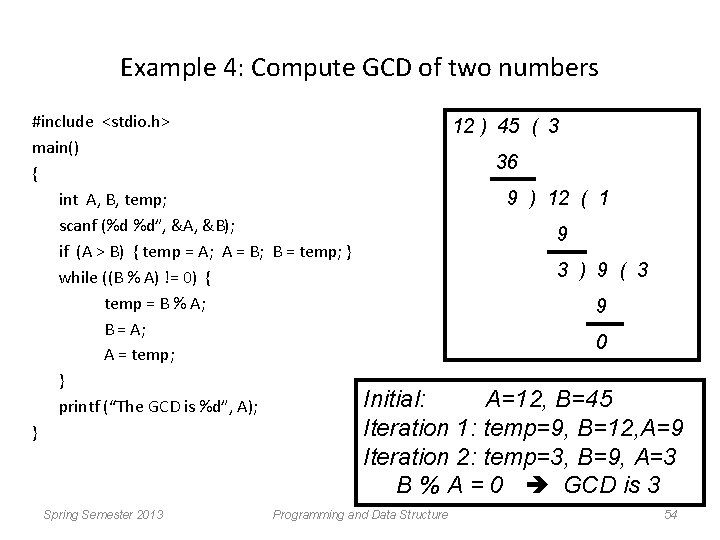Example 4: Compute GCD of two numbers #include <stdio. h> main() { int A, B, temp; scanf (%d %d”, &A, &B); if (A > B) { temp = A; A = B; B = temp; } while ((B % A) != 0) { temp = B % A; B = A; A = temp; } printf (“The GCD is %d”, A); } Spring Semester 2013 12 ) 45 ( 3 36 9 ) 12 ( 1 9 3 ) 9 ( 3 9 0 Initial: A=12, B=45 Iteration 1: temp=9, B=12, A=9 Iteration 2: temp=3, B=9, A=3 B % A = 0 GCD is 3 Programming and Data Structure 54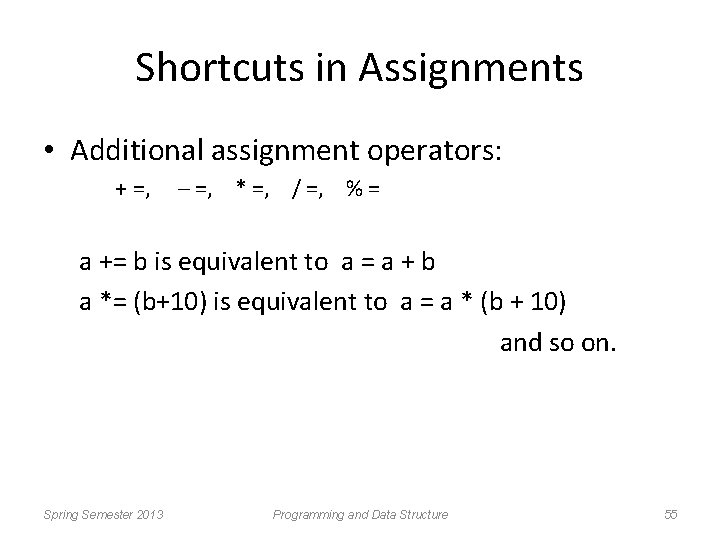Shortcuts in Assignments • Additional assignment operators: + =, – =, * =, / =, % = a += b is equivalent to a = a + b a *= (b+10) is equivalent to a = a * (b + 10) and so on. Spring Semester 2013 Programming and Data Structure 55More about scanf and printf Spring Semester 2013 Programming and Data Structure 56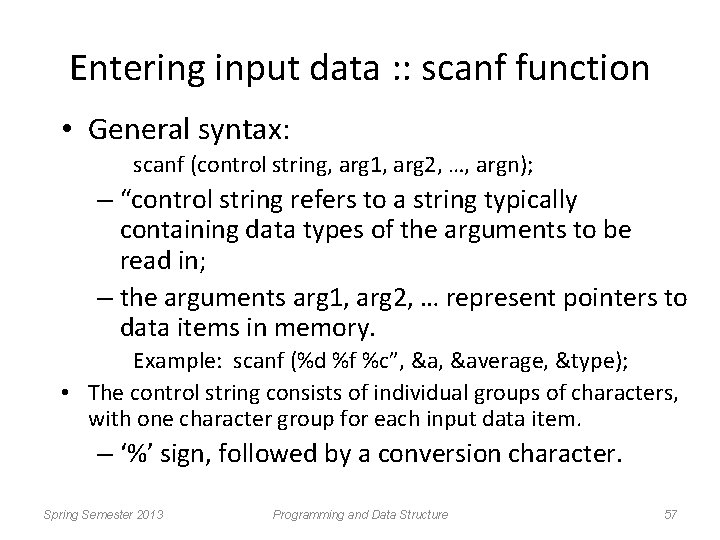Entering input data : : scanf function • General syntax: scanf (control string, arg 1, arg 2, …, argn); – “control string refers to a string typically containing data types of the arguments to be read in; – the arguments arg 1, arg 2, … represent pointers to data items in memory. Example: scanf (%d %f %c”, &average, &type); • The control string consists of individual groups of characters, with one character group for each input data item. – ‘%’ sign, followed by a conversion character. Spring Semester 2013 Programming and Data Structure 57– Commonly used conversion characters: c d f s X single character decimal integer floating-point number string terminated by null character hexadecimal integer – We can also specify the maximum field-width of a data item, by specifying a number indicating the field width before the conversion character. Example: scanf (“%3 d %5 d”, &a, &b); Spring Semester 2013 Programming and Data Structure 58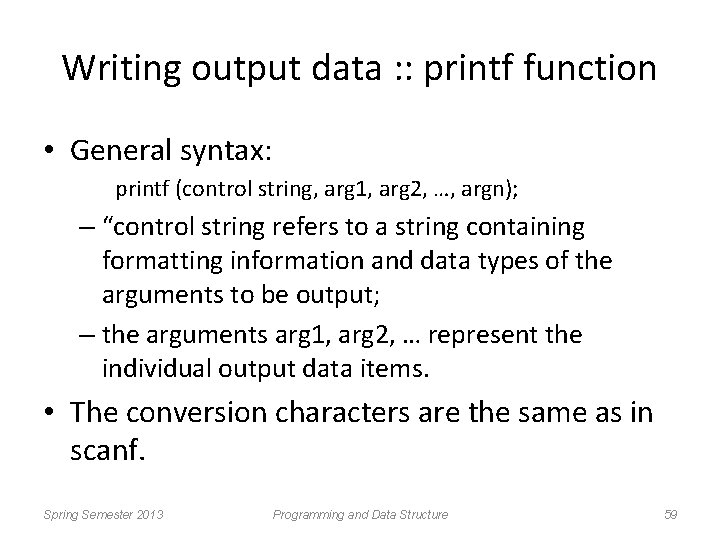Writing output data : : printf function • General syntax: printf (control string, arg 1, arg 2, …, argn); – “control string refers to a string containing formatting information and data types of the arguments to be output; – the arguments arg 1, arg 2, … represent the individual output data items. • The conversion characters are the same as in scanf. Spring Semester 2013 Programming and Data Structure 59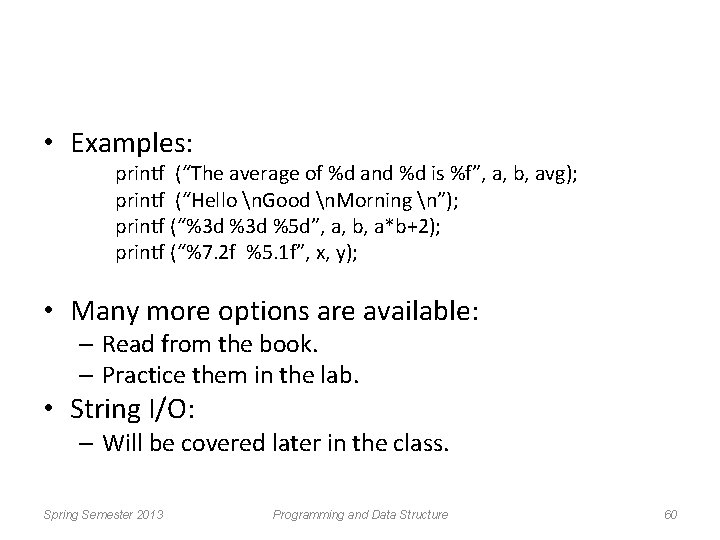• Examples: printf (“The average of %d and %d is %f”, a, b, avg); printf (“Hello n. Good n. Morning n”); printf (“%3 d %5 d”, a, b, a*b+2); printf (“%7. 2 f %5. 1 f”, x, y); • Many more options are available: – Read from the book. – Practice them in the lab. • String I/O: – Will be covered later in the class. Spring Semester 2013 Programming and Data Structure 60